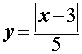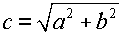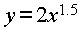# AP Computer Science Java: Lesson 1.5bMath Operations - Math Class Functions

Lesson 1.5b - Math Class Functions
Purpose: To learn how to use Java's Math class functions

The Math Class
So far we have used only basic math operations. Now we will see how Java provides for more complex math such as square roots and exponents. To perform these and other math functions (or methods), we will use Java's Math class. There are many math methods available, but we will only use the following. Note their use in code in the examples shown.

 Method What it does Example of use in code int abs(int x) Returns the absolute value of the integer parameter x int z = Math.abs(x+y); double abs(double x) Returns the absolute value of the double parameter x double z = Math.abs(x-y); double pow(double x, double y) Returns x to the power of y double z = Math.pow(x,y); double sqrt(double x) Returns the square root of x double z = Math.sqrt(y);

More Examples
To better illustrate the use of Math functions, let's look at some more examples.

Example 1: Suppose you wanted to create the following math equation:In Java, we would write

y = Math.abs(x - 3) / 5;
//NOTE: y must be a double if a decimal result is desired

Example 2: Suppose you wanted to create the following math equation:In Java, we would write

c = Math.sqrt(a*a + b*b);
//NOTE 1: c must be a double if a decimal result is desired
//NOTE 2: don't use Math.pow for an exponent of 2

Example 3:
Suppose you wanted to create the following math equation:In Java, we would write

y = 2 * Math.pow(x, 1.5);
//NOTE: y must be a double if a decimal result is desired